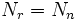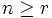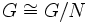# Ascending chain condition on normal subgroups implies Hopfian

This article gives the statement and possibly, proof, of an implication relation between two group properties. That is, it states that every group satisfying the first group property (i.e., group satisfying ascending chain condition on normal subgroups) must also satisfy the second group property (i.e., Hopfian group)
View all group property implications | View all group property non-implications
Get more facts about group satisfying ascending chain condition on normal subgroups|Get more facts about Hopfian group

## Statement

If a group satisfies the ascending chain condition on normal subgroups, then it is Hopfian: it is not isomorphic to any of its proper quotients.

## Definitions used

### Ascending chain condition on normal subgroups

Further information: Group satisfying ascending chain condition on normal subgroups

A group$G$ satisfies the ascending chain condition on normal subgroups if, whenever$N_i$ is a chain of normal subgroups of$G$:$N_1 \le N_2 \le \dots$

then there exists a finite$r$ such that$N_r = N_n$ for all$n \ge r$. In other words, the series stabilizes after a finite length.

### Hopfian group

Further information: Hopfian group

A group$G$ is termed Hopfian if$G$ is not isomorphic to$G/N$ for any nontrivial normal subgroup$N$ of$G$.

## Related facts

### Similar facts

• Artinian implies co-Hopfian: Artinianness is a descending chain condition on subgroups, and co-Hopfian is the condition of not being isomorphic to a proper subgroup.

## Proof

We prove the contrapositive here: any group that is not Hopfian does not satisfy the ascending chain condition on normal subgroups.

Given: A group$G$ that is not Hopfian.

To prove:$G$ does not satisfy the ascending chain condition on normal subgroups.

Proof: Suppose$N$ is a nontrivial normal subgroup of$G$ such that$G \cong G/N$ (the existence of$N$ follows from the assumption of not being Hopfian). Suppose$\varphi:G \to G/N$ is the quotient map and$\alpha:G \to G/N$ is an isomorphism (NOTE:$\alpha$ is not the quotient map).

Now, define inductively:$N_0 = 1, N_1 = N, \qquad N_{i+1} = \varphi^{-1}(\alpha(N_i))$.

Note that we have:$N_{i+1}/N_i = \varphi^{-1}(\alpha(N_i))/\varphi^{-1}(\alpha(N_{i-1})) \cong \alpha(N_i)/\alpha(N_{i-1}) \cong N_i/N_{i-1}$.

(the second equality here is by the fourth isomorphism theorem).

By the inductive assumption, we see that for all$i$:$N_{i+1}/N_i \cong N$.

In particular, the$N_i$s are a strictly ascending chain of subgroups.

Further, induction on$i$ shows that, since$N_i$ is normal in$G$,$\alpha(N_i)$ is normal in$G/N$, and thus, since normality satisfies inverse image condition,$N_{i+1}$ is normal in$G$.

Thus, the$N_i$s form a strictly ascending chain of normal subgroups, and hence,$G$ does not satisfy the ascending chain condition on normal subgroups.

### Alternative way of writing the above proof

If we define$\sigma = \alpha^{-1} \circ \varphi$,$\sigma$ is a surjective endomorphism from$G$ to itself. We can then alternatively defin$N_i$ as the kernel of$\sigma^i$, the$i$-fold composite of$\sigma$ with itself.